# Visual Representation of Matrix and Vector Operations and implementations in NumPy, Torch, and TensorFlow

September 23, 2020 • By Balakrishna Kumar

# 3. Array Initialization ~ 1-D, 2-D, 3-D

### Scalar and 1-D Array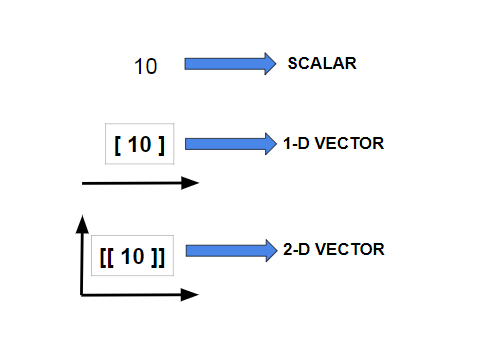Scalar, 1-D, 2-D arrays

### 2-D Vector Array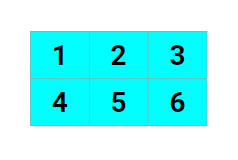2-D arrays

# 4. Generating Data

### Scalar and 1-D Array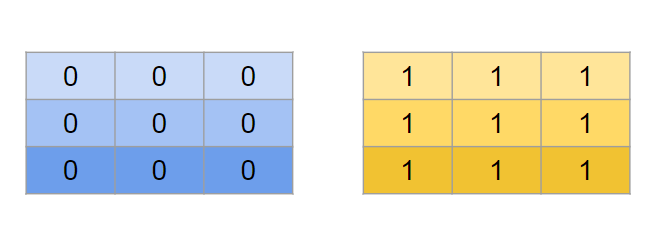Zeros and Ones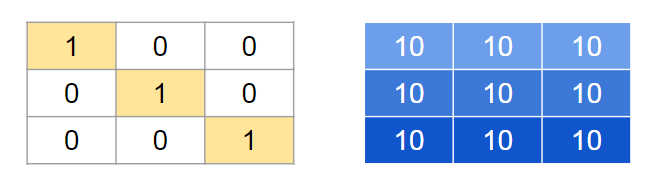Diagonal & Same element fill

### Draw random samples from the Normal distribution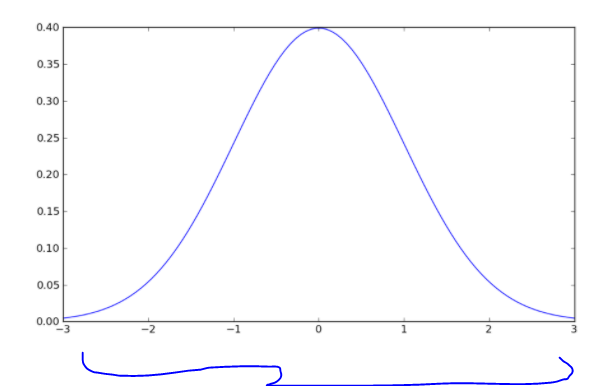Normal Dist’n Bell Curve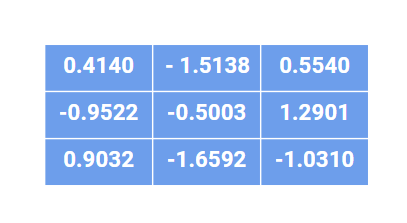Samples were drawn from Normal Dist’n

### Draw samples from the uniform distribution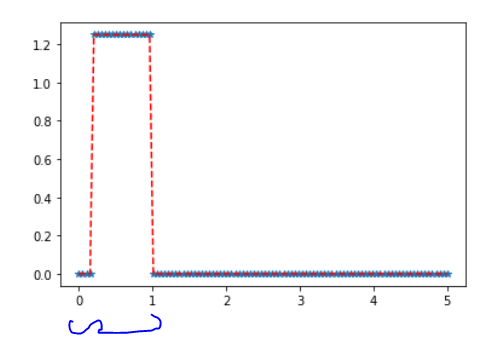Uniform Dist’n Curve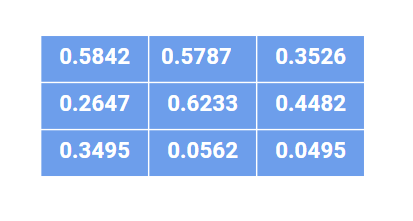Samples were drawn from Uniform Dist’n

# 5. Vector Arrangements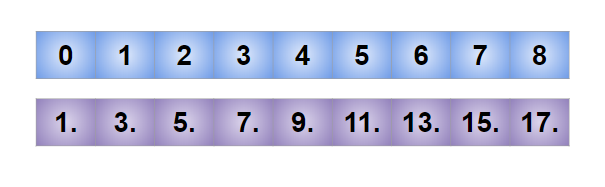Zeros and Ones

# 7. Math Operations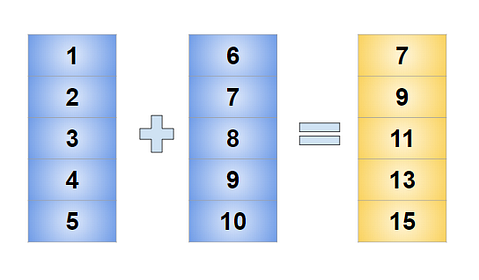Sum and Subtract operations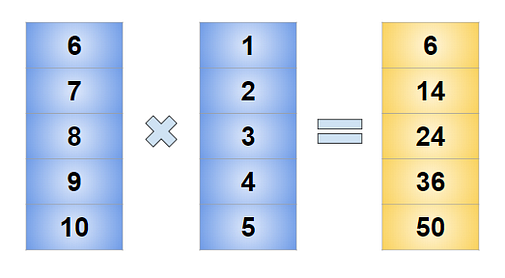multiply and divide operations

# 8. Dot Product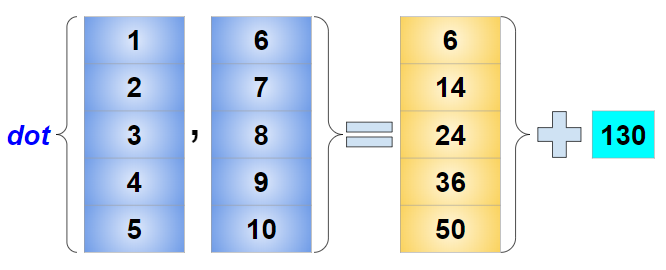Dot Product

# 9. Matrix Multiplication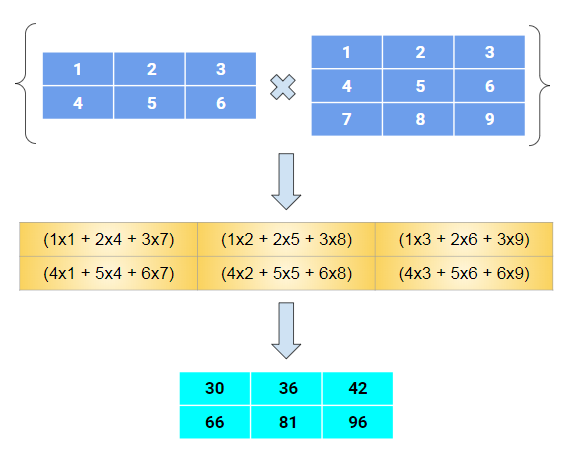Matrix Multiplication

# 10. Indexing and Slicing (2-D)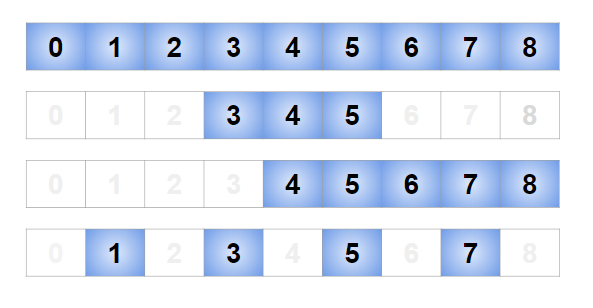Indexing and Slicing (2-D)

# 11. Indexing and Slicing (2-D — Matrix)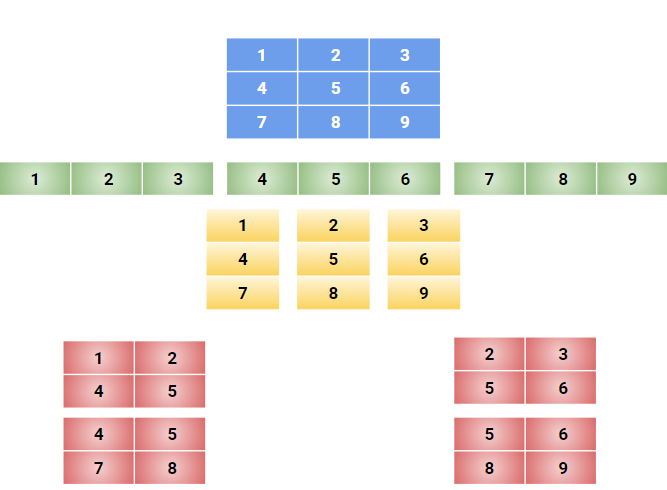Indexing and Slicing (2-D — Matrix)

# 12. Reshaping and Transpose axes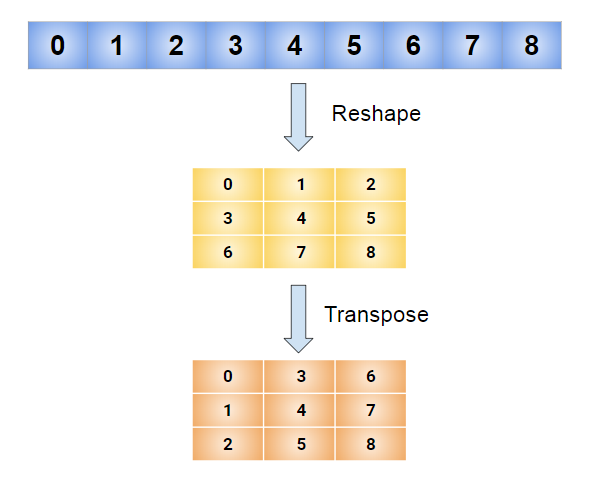Reshaping and Transpose axes

# 13. Concatenation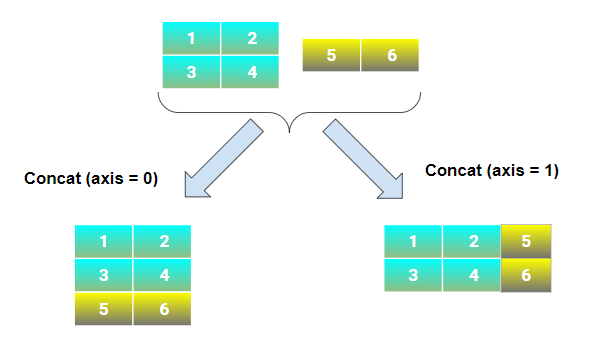Concatenation

# 14. Summing across axes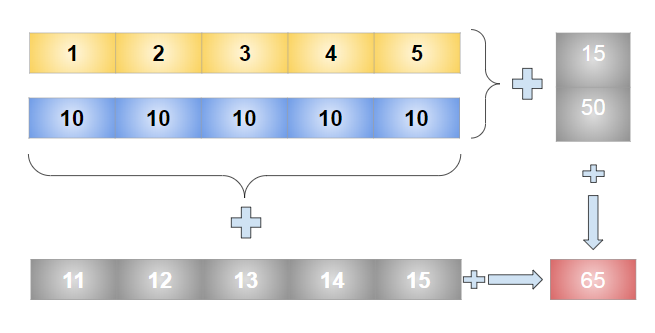Summing across axes

# 15. Mean across axes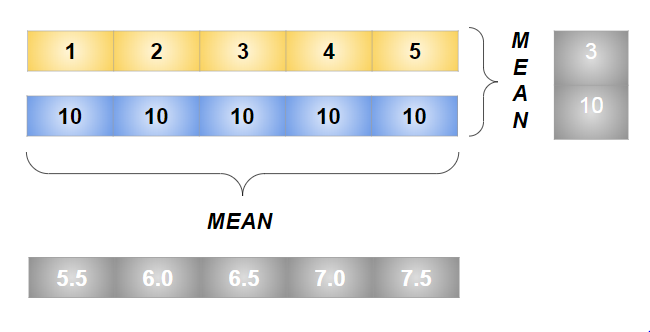Mean across axes

# 16. Dimension Expansion & moving axes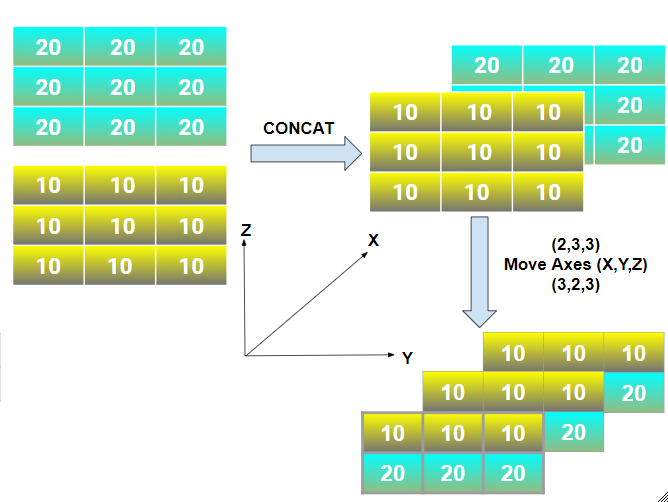Dimension Expansion & moving axes

# 17. Max (Min) and Argmax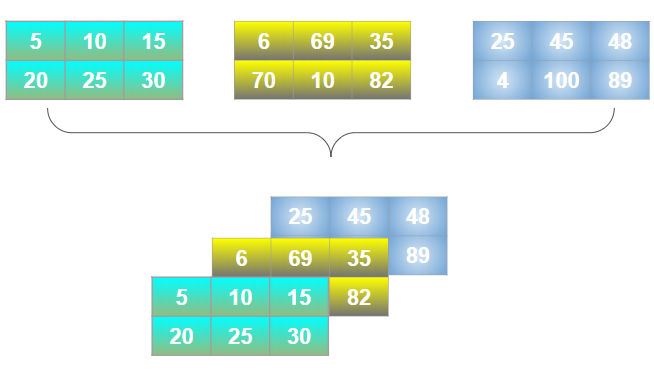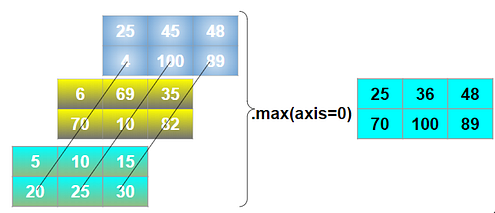Max for axis=0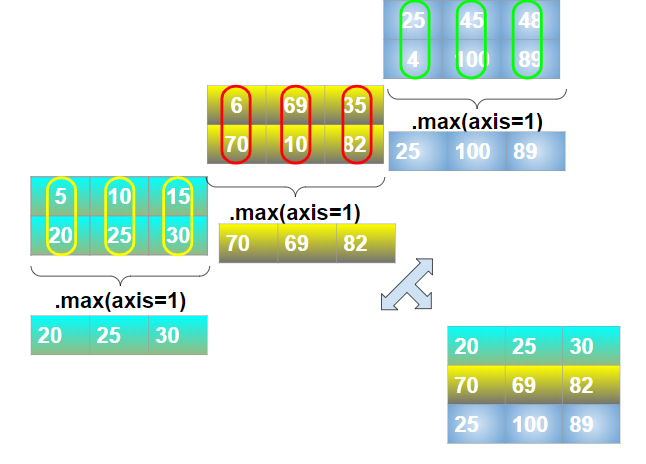Max for axis=1

# 18. Slicing and Indexing (3-D Matrix)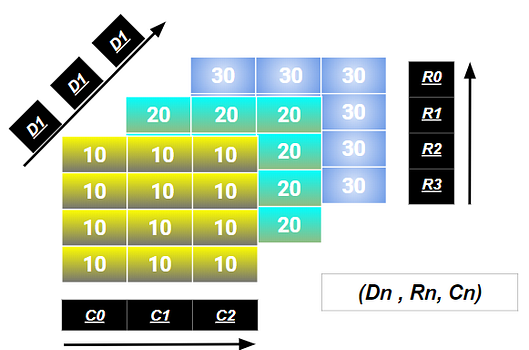3x3 Matrix and its indices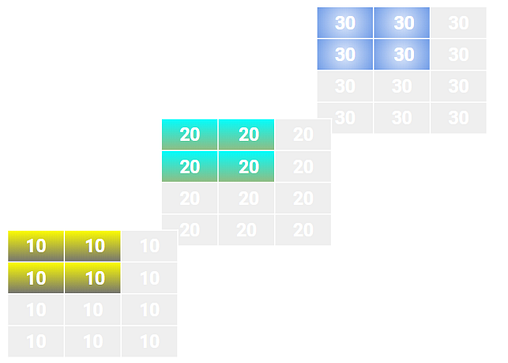Upper-Left & Lower-Right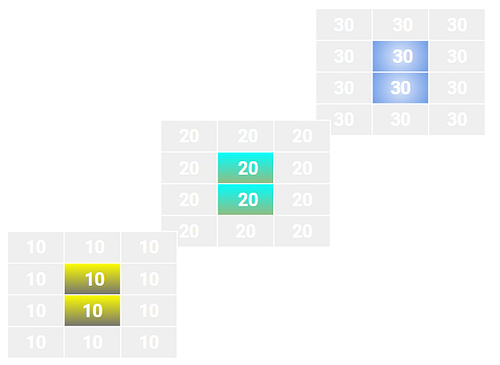Middle Elements & Inverse Middle Element

### Torch Implementation:

Due to visualization constraints, I skipped the operations on the higher dimension parts.

I hope I was able to provide some visual understanding to some of the fundamental operations along with your choice of deep learning framework and I will add more detailed operations shortly.

Check out the Notebooks that contains the entire code implementation and feel free to break it.

Complete Code Implementation is available at,

Until then, see you next time.